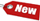Browse Accounting Lessons HereAccounting Terms & DefinitionsAccounting for Merchandising ActivitiesDebits and Credits (Double Entry Accounting)Business Valuation FormulasTime Value of Money & Present/Future ValuesComplex Debt & Equity InstrumentsCommon Stock & Shareholder's EquityAccounting & Finance RatiosValuing Common StockCorporate Income TaxesLower of Cost or Market (LCM) & Inventory ValuationChart of Accounts & BookkeepingBonds Payable & Long Term LiabilitiesCapital AssetsGAAP, Accrual & Cash Accounting, Information Commodity, Internal Controls & MaterialityWhat category of browser are you on this website? Accounting student (homework help) Finance professor (university research) Accounting manager (at work) Other Explore Careers in Accounting and FinanceVisit our section on Careers in Accounting & Finance to explore vast opportunities in this industry.

Chapter 4.3® - How to Use a Financial Calculator BAII Plus to Perform Time Value of Money & Present / Future Value Calculations

The future value calculations we did above can be very tedious when say the investment is set to grow not over 3-5 years, but over 30-35 years. Is there an easier way to perform these calculations? Yes there is! Your financial calculator can help make these calculations a breeze, but learning a financial calculator is very tricky at first, but very much fun after you learn it! In this section, we will show you how to perform present value/ future value calculations on your financial calculator. We will be using BA II Plus Texas Instruments Financial calculator (Model# S-1205D).

Financial calculators have the competitive advantage of handling lots of computations but that is pretty much all they can do. In other words, you as the financial/business analyst must understand the problem at hand and be able to program the financial calculator to achieve your desired answer.

 PV = Present Value FV = Future Value N = Number of periods, what we have called “t” in our future value equation i = Interest rate, which we have called “r” in our future value equation. PMT = Payments per period BGN = Beginning of Period Calculations C/Y = Compounding of interest per period P/Y = Number of periods per year

Practice ProblemLet’s solve a time value of money question by using our financial calculator. Referring to our original example of \$100 invested at 10% annual interest rate, what will we have in 5 years, assuming the interest rate does not change?
Original Problem: Consider you invest \$100 in a savings account that pays 10% interest per year, how much will you have at the end of the 5 years?

 Steps 1) Enter -100. Press the PV key 2) Enter 5. Press the N key. 3) Enter 10. Press the %i key (notice we enter 10% as “10” and not 0.10) 4) To calculate the future value, select CPT, then select FV.5) You should get an answer of \$161.051

To make it easier to understand the calculations, make a table like below:

 5 10 -100 N %i PMT PV FV? Solve for FV: \$161.05

Guidelines for Using a BA II Plus Financial Calculator

i) Before you perform any new calculation, clear out the calculator as this is very important. If you do not clear your calculator, you will get an answer based on information input earlier, which will most likely provide you with false answers. In order to clear the previous values in the calculator, press the 2nd key, then CLR TVM. CLR TVM stands for Clear Time Value of Money. Also note that turning off the calculator and turning it back on won’t work, you must clear the previous histories. Also if you are in the middle of a calculation and make a mistake, clear it out and start over. It’s better to be safe and correct, than doubtful of your calculations and ultimately sorry for the wrong answer!

ii) Put a negative sign on cash outflows. Be sure to put a negative sign on cash outflows (outgoing payments), and a positive sign on cash inflows (incoming payments). This also means you should enter the present value amounts with a negative sign because the present value represents the amount you are giving up today in exchange for cash inflows later. No wonder in our example above, we set the present value at \$-100 at 10% interest for 5 years, to arrive at a positive \$161.05 future value answer.

iii) Enter interest rates correctly. Financial calculators assume that interest rates are quoted in percent, thus if the rate is 0.10 or 10%, you would input this simply as “10” in the calculator.